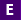#Not a Florida public school educator?

Access this resource
on CPALMS.com

### General Information

Subject(s): Mathematics, English Language Arts, Science
Intended Audience: EducatorsSuggested Technology: Computer for Presenter, Computers for Students, Internet Connection, Interactive Whiteboard, Adobe Acrobat Reader
Instructional Time: 40 Minute(s)
Keywords: slope, slope intercept, slope intercept form, proportional relationship, unit rate, y intercept, scratch, scratch program, linear relationship, scratch, program, coding, computer science, computer, plot the graph, graphing, flow chart
Instructional Component Type(s):

## Aligned Standards

This vetted resource aligns to concepts or skills in these benchmarks.

## 2 Lesson Plans

Slope Intercept - Lesson #1

This is lesson 1 of 3 in the Slope Intercept unit. This lesson introduces graphing proportional relationships. In this lesson students will perform an experiment to find and relate density of two different materials to the constant of proportionality and unit rate.

Slope Intercept - Lesson #3

This is lesson 3 of 3 in the Slope Intercept unit. This lesson introduces similar triangles to explain why slope is the same between any two points on a non-vertical line. In this lesson students perform an activity to determine that slope is constant throughout a line and students will discover the slope for vertical and horizontal lines.

## Related Resources

Other vetted resources related to this resource.

## Lesson Plans

Slope Intercept - Lesson #3:

This is lesson 3 of 3 in the Slope Intercept unit. This lesson introduces similar triangles to explain why slope is the same between any two points on a non-vertical line. In this lesson students perform an activity to determine that slope is constant throughout a line and students will discover the slope for vertical and horizontal lines.

Type: Lesson Plan

Slope Intercept - Lesson #1:

This is lesson 1 of 3 in the Slope Intercept unit. This lesson introduces graphing proportional relationships. In this lesson students will perform an experiment to find and relate density of two different materials to the constant of proportionality and unit rate.

Type: Lesson Plan